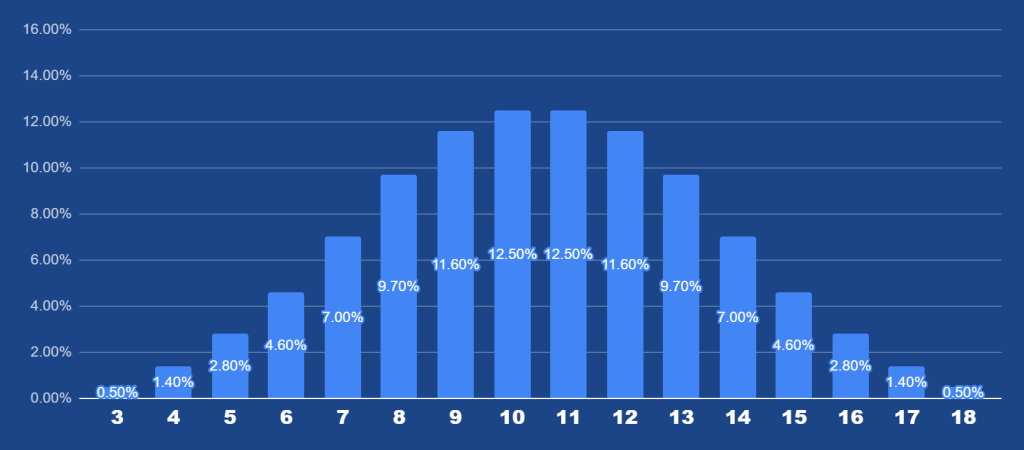# Lightning Dice from Evolution: Probabilities and How to Play

Exclusively from Evolution Gaming, Lightning Dice is a game that has struck hard in the hearts of the Game Shows crowd. Three Dice, of face value 1-6 are dropped into a twisting and turning transparent “ Lightning Tower”, watching them fall through to the bottom of the maze and landing on 3 values respectively. This game promises unlimited entertainment is quite simply, very addictive. You may find yourself chasing those huge payouts, but we suggest you take it slow. Get a feel of the game, and then bet on probability.

## The Rules

Players can bet on 16 spots, 3-18. These numbers are simply the sum of the outcome of all 3 dice. So for example, if the three dice land 3,6 and 2, the spot that wins would be 3+6+2=11. Each spot has a different payout ranging from 5x to 150x.

But wait, it gets better. It’s a Lightning Game after all. At the beginning of every roll, the Game presents bonus lightning strikes. When the betting is closed, 2-3 spots are randomly chosen for the special bonus x payout that can reach as high as 1000X! These are random and no particular pattern is followed in determining the Lightning payout spots.

## Payouts

The bets you’re allowed range from €1 to €2000.

Sum of all dice : x(bet amount) payout.

 Number Multiplier 3 50x 4 50x 5 25x 6 15x 7 10x 8 7x 9 6x 10 5x 11 5x 12 6x 13 7x 14 10x 15 15x 16 25x 17 50x 18 150x

So if you were to bet €1 on 18, you would win €150!

### Probabilities

The RTP for this game is at 96.21%, based on total 3 or 18 bets.

Three 6 sided dice are used in this game. Here, we will show you the probability of each outcome happening:• Probability of a sum of 3: 1/216 = 0.5%
• Probability of a sum of 4: 3/216 = 1.4%
• Probability of a sum of 5: 6/216 = 2.8%
• Probability of a sum of 6: 10/216 = 4.6%
• Probability of a sum of 7: 15/216 = 7.0%
• Probability of a sum of 8: 21/216 = 9.7%
• Probability of a sum of 9: 25/216 = 11.6%
• Probability of a sum of 10: 27/216 = 12.5%
• Probability of a sum of 11: 27/216 = 12.5%
• Probability of a sum of 12: 25/216 = 11.6%
• Probability of a sum of 13: 21/216 = 9.7%
• Probability of a sum of 14: 15/216 = 7.0%
• Probability of a sum of 15: 10/216 = 4.6%
• Probability of a sum of 16: 6/216 = 2.8%
• Probability of a sum of 17: 3/216 = 1.4%
• Probability of a sum of 18: 1/216 = 0.5%

As you can see above, the probability of 3 and 18 rolling is the least, at only 0.5%. Hence, the massive 150x payout. Not to mention if a Lightning for 1000x strikes on 3 or 18, you’re on your way to buying a Villa in Las Vegas!

The most probable outcomes are the middle spots: 10 and 11, followed by 9 and 13, and so on.

There is no particular strategy to play this game. So we recommend playing around the different spots, sticking to the middle if you’re a safe player, and have tons of fun. Check out Lightning Dice at LeoVegas to try your own luck!LuckyLunaTVZeethBrazucassinoLetsGiveItASpin